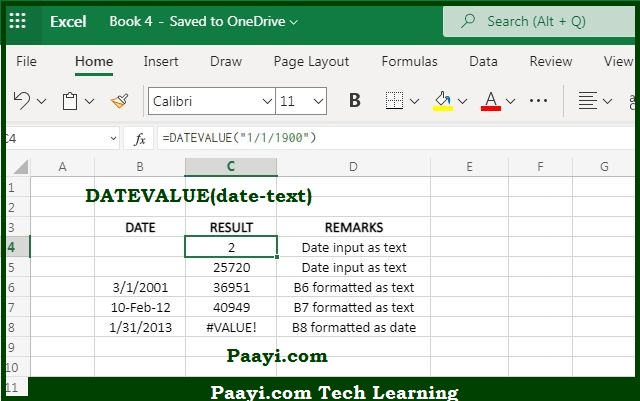# Learn How to Use Microsoft Excel DATEVALUE Function

Written by | 0 Comments | 829 Views

In this article, you will learn how to use the Microsoft Excel DATEVALUE function and its prime function in Microsoft Excel. You will also get to know the Microsoft Excel DATEVALUE function return value and syntax with the help of some examples.

## Microsoft Excel DATEVALUE Function

The main job of the DATEVALUE function is to convert a text format into a valid date. That means the Microsoft Excel DATEVALUE function converts a date which is represented as a text in the proper Excel date format.

You can understand this by this example - the formula = DATEVALUE("12/31/2020") will return a serial number according to the Excel date system, which will represent - December 31, 2020. The proper Excel dates are more useful in worksheets than text dates because you directly manipulate them using formulas & pivot tables to create summaries and perform various date-related analyses.

### DATEVALUE Function Return Value

It will return a serial number that represents a specific date as per Excel.

### DATEVALUE Function Syntax

= DATEVALUE(date-text)

Where:

• date-text: Any valid date provided in the text format.

## How to Use Microsoft Excel DATEVALUE Function?In case date-text is the address of the cell, the value of the cell must be the text. If you want to enter the date-text directly into the formula, you should enclose it in the quotes. If it happens that you have referred a date-text to a cell that does not contain a date formatted as a text, then you will get the return result as a #VALUE error.